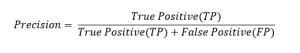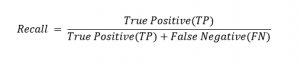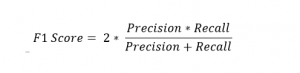Blogs/Precision and Recall

# Precision and Recall

blazedafyah Jul 02 2021 2 min read 192 views

NIT Warangal graduate, Software Engineer at Wipro.

FundamentalsThe performance of classification models is often measured by the accuracy score: the number of correct predictions over the total output size. It is simple and straightforward, making it broadly useful. However, accuracy scores can be misleading in certain situations: imbalanced classification problems where one class represents the overwhelming majority of data points.

In such cases, a model that classifies all the data points as belonging to that class will achieve a very high accuracy score due to there being so few misclassifications. Precision and recall are two metrics that help better understand the ability of the classifier to correctly classify items in an imbalanced dataset.

Precision measures the ability of a model to identify relevant data points. In the simplest terms, it is the ratio between the True Positives and all the Positives.Intuitively, in such classification problems, the focus should also be on identifying the data points not belonging to the majority class. Or in other words, to correctly identify true positives. This is called Recall.To fully evaluate the effectiveness of a model, both precision and recall have to be evaluated. One ideally aims for high precision and high recall. However, both are not possible at the same time. Improving one comes at the expense of the other.

Therefore, a tradeoff has to be made between the two. The recall is to be maximized when False Positives should ideally be avoided (eg. classifying a patient as healthy when he is not actually). The precision should be maximized when False Negatives are to be avoided (e.g. classifying an important mail as spam).

There are situations where prioritizing one over the other is not an option. In such cases, the F1 score is used. F1 score is the harmonic mean of precision and recall.Precision and Recall both are very important metrics in evaluating classifiers. Finding a balance between them is very important to get the best possible model.

Learn and practice this concept here:

https://mlpro.io/problems/precision-and-recall/